Select Page

The Great Pyramid is not what you have been told, nor is its origin.  It is, though, full of enlightenment.

# The Pyramid of the Sun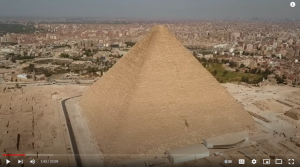We have seen the cosmic blueprints for a special 4-sided pyramid. It is aligned with the dimensions of, and relationships between, the Earth, Sun, Moon, and our entire Solar System. It unfolds from the simplest of parameters based on the 5 Essential Elements of Creation hidden as specific sets of Triplets within the Torah combined basic geometry. In this article we will show that not only did those Cosmic blueprints exist, but the plans were executed in ancient times. The Pyramid was not only built, but still stands today after thousands upon thousands of years, exactly where the Torah says it does.

After a brief recap of the blueprints for the Pyramid of the Sun, we will overlay them with the 2.3 million stone block Pyramid, and then break that down even further, illustrating even more cosmic and hyperspace alignments.

There is no pyramid of that size and stature mentioned in the Torah or anywhere in ancient texts or works of art. We have a secondhand reference to the pyramids of Egypt by Herodotus 400 BCE or so, yet despite some fine artwork going back 2500- 3000 BCE nothing about any pyramids. This intricate stone bas-belief of a massive ship was done around 2450 BCE in 5th Dynasty Egypt, so the Egyptians were very capable of capturing great details in their daily lives back then. So how did they miss the Pyramids? Another question arises as to why Pharoah did not go after the Israelites on his massive ships? Maybe our understanding of the timing and accuracy of history is a little off.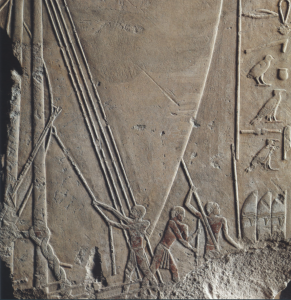Were the pyramids buried under the desert sands for millennia until the time of the First Temple. All we know is that in 820 CE caliph Abdullah Ma’moun blasted one open only to find nothing inside it but empty chambers and passageways, not even carvings or markings of any kind. Are the timelines of history all mixed up? In a Divine Simulation none of this would matter, as the Creator could insert anything into the simulation at any time, even a new backstory of history that we would have no choice but to believe. He would not even have to do this through micro-management as the program could be coded to do this automatically when so triggered, like AI inserting its own content in our TikTok feeds.  We would never know.

### The Torah Drops a Few Clues.The purpose of the Torah is not to serve as a history book. It is to guide us and help us find our way back to the truth. The Creator knew Man would lie and rewrite history to his convenience whether he had a book or not so the truth had to be concealed and preserved if man would ever be able to escape the illusion.

In such a manner, the Torah drops us a few definitive clues about a pyramid.  The word “bricks” is mentioned twice only in the Torah. The first time is in in Genesis 11, where it says, “The entire Earth had one language with uniform words,” and two verses later, “They had bricks to use as stone and mortar.” The Torah is always deliberate in its word choice and takes a myriad of equations and purposes into account with each one. For example, the numerical value of the word used for mortar, chemar (חמר) is 248 as in the 248,000φ quantitative elements in the Torah and so many key aspects of the Torah, like its 248 columns, and in atbash (reversed) gematria (סיג) has the numerical value of Chochma, the 2nd highest sefira (dimension) meaning Wisdom. In this form it means dross or the stoney waste metal slag produced from it. It can also mean fence or border and is also used twice in the Torah, to mean trespass.

Complementing it, the word for bricks, l’venim (לבנים) has a complete numerical value with the kolel of 756, as in “sefirot (ספירות). It is also the exact area of a cubit (27.5” x 27.5”), which is the same as the radiated Primal Frequency, (27.5 Hz)2 and thus the base measure of the Pyramid of the Sun (756 ft).

Because the word brick l’venah (לבנה) is the same as the Hebrew for the “Moon l’vanah (לבנה)” and comes from the word “white lavan (לבנ)”, we can extrapolate that the bricks were white. The only other place that bricks are mentioned in the Torah is in Egypt when Pharaoh tells Moses the Israelites will have to make bricks without straw.

Now, in Genesis 11, paragraph 32, the One World folks were building a tower to breach the divide between Heaven and Earth, to metaphysically invert the 11 sefirot and the 32 Paths between them to put physicality over spirituality, to replace Chochma (Wisdom) with the dross or slag, the world of illusion and suffering.

The specific word used there in the Torah for tower was Migdal (מגדל), and it is used twice there.  The word tower, Migdal (מגדל) is only found 5 times in the Torah, and it is once again found twice when the Israelites were escaping Egypt and camped before the Red (Endless) Sea, between the Tower Migdal (מגדל) and the Sea.

The bricks and Tower are thus tied together and while we know the Tower of Babel and the Cosmic or virtual Tower of Truth and Pyramid of the Sun are 210 cubits in height, the same as the ordinal value of the phrase from Exodus 14, “Camp before…Pi Charoth (Freedom Valley), between Tower and the Sea (פִּי הַחִירֹת, בֵּין מִגְדֹּל וּבֵין הַיָּם).” It is the same as the exact height of the Great Pyramid in Giza, 210 cubits comprised of exactly 210 levels, and they all match and align with the 210 years of the Israelite exile in Egypt, just in case there was an doubt as to which Tower was being referred. Moreover, the complete value of between Tower and the Sea (בֵּין מִגְדֹּל וּבֵין הַיָּם)” is 380, that of the word Mizraim, meaning Egypt. And while the ordinal value of Migdal (מגדל) is 32 as in paragraph 32, its revealing atbash gematria (ירקכ) value begins with 210 and has a full value of 330, exactly like the height and base measurements of the Cosmic (virtual) Pyramid of the Sun and of the Great Pyramid in Giza, which is found at 30o latitude, like the ordinal value of its first two letters (יר) , second two letters (קכ), and the value of its first and last letters (יכ).

Moreover, “Tower, Migdal (מגדל)” has a numerical value of 77, whose square root is 8.775 or 5778 in reverse, and while this was the 3rd of the 42 Journeys described in verse 33:7 of the Book of Numbers we know that the 11 Triplets of Creation is comprised of the Torah’s first 33 letters that equal 3003, the 77th Triangular Number. We also know that this occurred in 2448 HC, 3330 years before 5778, after the 210 years of exile in Egypt.

The value 330 is also that of the first 3 letters of “Mizraim (מצר), Egypt,” which is also the 60th Triplet (מצר) in the 72 Triplet Matrix, matching the total ordinal value Migdal’sl (מגדל) revealing atbash gematria, 60.

The word Egypt Mizraim (מצרים) is found 363 times in the Torah, as in the value of H’Moshiach, Cosmic Consciousness, and as in the Spherical Time circumference 36304.24470, and as in the Great Pyramid’s perimeter, 36300 inches. Of those 363 times, 33 times it is as “to/in Egypt Mizraim (במצרים)” leaving 330 other times without that special 42 (במ) distinction.

### Complementary Concepts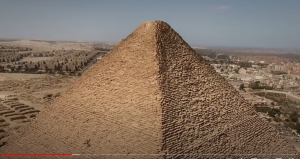Meanwhile, while the word value of the Pyramid’s base (330) is found 86 times in the Torah, as in Elohim (אלהים), the word value of the Pyramid’s apothem 267 is found 21 times in the Torah, as in the higher Name Ehyeh (אהיה). Together they are found (86 + 21) = 107, as in the 107th Triangular Number, 5778, the Spherical Time radius and Event Horizon. It also relates to the 107,000th letter after Bereshit, at the start of the 10 Commandments, which are complementary to the 210 years in Egypt. Meanwhile, the 107th time the word “Egypt Mizraim (מצרים)” is found in the Torah is, like the 10 Commandments, at Mt Sinai, at the burning bush, when the Creator says for the first time, “…when the people leave Egypt,”

All numbers have complements that make them whole to specific round numbers (concepts) like 1000 that represents completeness on the level of Binah. So, we see that 380 is balanced with the complementary 620 of Keter, the highest crowning level, as in the 620 letters of the 10 (י) Commandments that connect to keter in numerous ways.  Another connection between the value of Egypt, 380 and both the Torah and Keter is found in the 304,805 letters in the Torah. Exactly 4805 of them are the letter Pe (פ) of numerical value 80, leaving precisely 300,000 of the other 21 letters. Since the letter Shin (ש) is both the 21st letter and has the value 300, the 304,805 letters represent the letters Shin (ש) and Pe (פ), which together are 380, like Egypt. Those 4805 occurrences of the letter Pe (פ) have a total numerical value of (80 x 4805) = 384,400, which not only aligns with the 384,400 kilometer distance between the Earth and the Moon, but with Keter, as it is exactly 6202.

Meanwhile, the complementary value to the word “In Egypt Mizraim (במצרים)” is 618, which splits 1000 into the perfect Phi (φ) and 1/φ2 proportions. “In Egypt Mizraim (במצרים)” begins with 42 (במ) like the 42 Israelite journeys that began immediately after the death of the first born whose initials are 42 (במ) comprising the final initials of the 10 plagues, whose 11 initials total 541, the value of “Israel.” The rest of the letters in “In Egypt Mizraim (במצרים)” have a value of 340, as in the word, Shem (שם), so the entire word numerically translates to Shem MB or the 42-Letter Name.

Like the word “Egypt Mizraim (מצרים),” the Hebrew word for “Pyramid (פירמידה)” begins with the numerical value 330 (פירמ), and both words contains 210 (יר). Pyramid (פירמידה) also contains “Pad, redemption (פד),” of numerical value 84, as in the two 42 Letter Names. And while, “Pi Charoth (פִּי הַחִירֹת)” has a valuation with the kolel of 720, and while it begins with the same two letters (פי) as “Pyramid (פירמידה),” it ends in (חִירֹת) which contains the 27th Triplet (ירת) of the 72 Triplets, and has the same numerical value (618) as 1/φ, the complement to “In Egypt Mizraim (במצרים)” of numerical value 382.

For those that understand and make the annual switch in the daily prayers from “rain geshem (גשם)” to “dew, tal (טל)” in Pesach and Sukkoth, together their value is also (343 + 39) = 382.

### Tower of Truth and the 10 Commandments

In creating a virtual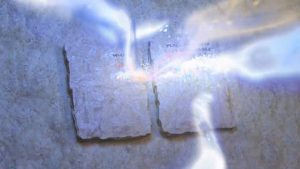Cosmic Tower of Truth, we began with the separate 620 letters of the 10 (י) Commandments in Chapter 20 of Exodus. We know that the 27 Hebrew letters are derived from the 22 Names of those letters and that the Name for the letter Yud (יוד) has a value of 20. The Hebrew word for 20, esrim (עשרים) has a numerical value of 620, as in the Hebrew word Keter, the highest of the sefirot, their summit, equaling the value of the 62 Yuds (י) in the 10 (י) Commandments. This is why the Tower of Truth has 20 stages consisting of 20 sequentially stacked cubes (13 + 23 + 33203), giving rise to its (1 + 2 + 320) = 210 cubit height, and its 44,100 cu3 volume, and since 441 is the value of the Hebrew word Emet (אמת) meaning truth, hence the name “Tower of Truth.”

Like the 10 Commandments that begin with the 420th YHVH (יהוה) in the Torah, the 20 stages also align with the YHVH (יהוה) of numerical value 26 in that the precise sum of the logarithms of 26 in all 10 bases equals 19.99799 or 20.

Several key numbers have a similar precision in summing up their 10 bases close to a whole number, but none more than the precise sum of the logarithms of 937 in all 10 bases that equals 41.9997 or 42. The second closest number is the precise sum of the 10 logarithms of 12700 for all 10 bases, which is 57.9995758 or (100 42).

Like the word for Pyramid (פירמידה) and the name of Pi Charoth (פִּי הַחִירֹת) that contain the value 210, the isolated 13 verses of the 10 (י) Commandments have a total of 210 empty spaces within them, and while their (620 letters, 13 verses, and 172 words) = 805, so does the complete value (805) of Pi Charoth, Freedom Valley, (פִּי הַחִירֹת), and so does G-d’s Covenant with the Earth after the Flood, “the rainbow (הקשת),” whose ordinal value is 62.

### The Tower of Truth Becomes a Pyramid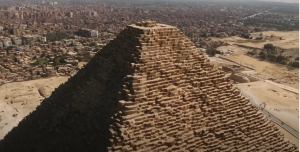We also had to establish a measurement for the cubit and the most logical one was to base it on the Primal Frequency 27.5, and make it 27.5” because this is the only measure that would align it with the deepest aspects of the Cosmos and the aether.

As we know from our studies on the 10 Commandments, the value 20 represents the crowning dimension of Keter. The 20 stacked cubes of the Tower of Truth at the core of the Pyramid add up to exactly 210, but they integrate with Phi (φ) to define it as well, and to define its base too.  The Tower and the Pyramid are 210 levels and 210 cubits tall, and 20 x 27.5(1 1/φ) = 210.0, which is off from 210.09826, as in the 21009826 total gematria of the Torah, by only .008%.

The base of the Pyramid is approximately 756 feet or 20 x 27.5(2 – √φ).  We should keep in mind that the letter Caf (כ) of numerical value 20 is the letter that is not only in the middle of the exponential curve of the alef-bet, but also stands out as the one that is precisely on that curve.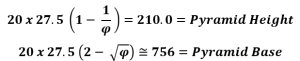From the height of 210 cubits, we made a simple right triangle using 722 or 51.84o as our angle of ascent because the 72 Triplets were used alongside the 42-Letter Name in the splitting of the Red Sea associated with the Tower, Migdal (מגדל), and with Pi Charoth (פִּי הַחִירֹת) and because the value 42 was already incorporated in the design as (5 x 42) = 210, like the 5 times the Tower, Migdal (מגדל) is mentioned in the Torah including during the 42 Journeys.

As it turns out using 72 and 42 gave us a Triangle that forms a perfect Pythagorean Phi (φ) triangle.

Those choices and Pythagoras’ theorem gave us the 165-cubit base, which just happens to align with the mod (27.5) 20 number cyclical repeat of the Fibonacci Numbers that always totals 165.

Flipping or mirroring that right triangle to form an isosceles triangle gave us the full base measure of (2 x 165) = 330 cubits. Then simply creating a similar triangle perpendicular to the first and squaring off the base gave us a perfect pyramid whose corner angles are 42o and whose face to face angles are 112o, and whose apothem is 267 cubits.  It was all back of the napkin stuff. Everything unfolded by itself.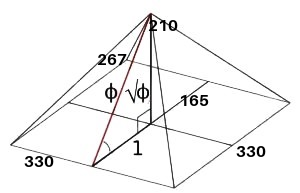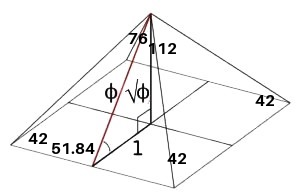And yet, the Great Pyramid’s height is also 210 cubits; its base is also 330 cubits; its base is scored in the middle at exactly 165 cubits; its ascending angle is 51.84o; its corner angles are 42o; its face to face angles are 112o, and its apothem is 267 cubits.  The base of the Great Pyramid though is not only 330 cubits but is 756.25 ft, which is both 27.52 and (12 x 27.5). And its height is 481.25 ft, which is (210 x 27.5”), meaning the builder of the Great Pyramid used the same cosmically derived cubit as we chose.

### Golden Ratios

The Phi (φ) angle built into the Pyramid is known as the Golden Ratio, but there is even more gold hidden in the Pyramid ratios. The ratio of the Pyramid’s base to its height whether in cubits, feet, or inches is exactly (330/210) = 1/.636363 = 1.571430, and 1571 is the 248th Prime Number, central to the Torah in so many ways, and hidden in the mortar. The height/base ratio (1.571430) is also one-half of 3.142860, a close approximation to Pi (π). We have already covered the value 3142 extensively because it is so important as the small gematria of “I am that I am (אהיה־אשר־אהיה)” found at Exodus 3:14 and as the numerical value of the 3 iterations of the Names Ehyeh (אהיה) and YHVH (יהוה).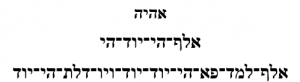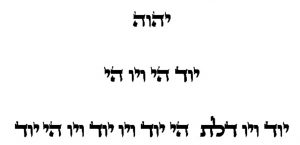It is also the difference (3.1428) between the atomic weights of gold, silver, and copper—the 3 metals of the Torah (368.3988—and the number of days in sidereal year, 365.256, or (368.3988365.256) = 3.1438. We have previously connected those 3 metals and their atomic weights to the Alchemist Stone at the central summit platform of the 72 Letter Triplet Matrix and now we can add that the Matrix total value divided by the 3 metals is 9143/368.3988 = 24.8182, as in Abraham (248) and as in Jacob (182). It is notable the word for mortar and covertly dross and/or slag is part of the Cosmic and metaphysical refinement of the 3 precious metals.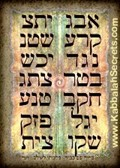We find the Cosmic and Divine value 3142 again appropriately as 7.3142, the result of dividing the 42-Letter Name Matrix by its 1st level, 3701/506 = 7.31422, and yet again by dividing 9 by that same first level in small gematria, 9/.123049 = 73.142, or more accurately, 73.141593, as in Pi (π).

Nonetheless, when we cross between the 3 measuring units and divide the base in feet by its height in cubits, we get 756/210 = 3.60 as in the 360o in a circle, which naturally is predicated on Pi (π).

What is interesting is that another cross measure of the height in inches divided by the base in cubits is 5775/330 = 17.5, as in the 175 years of Abraham (248), which aligns with the 248th Prime Number ratio (330/210). That is a lot of Cosmic cross-pollination! And even more outlandish is that Abraham is the 20th generation on the lineage of Adam as in the 20th Triangular Number, 210, and the Tower of Truth.

### Rainbows over Giza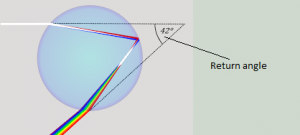The 42o corner angle of the Pyramid is not only an allusion to the 42-Letter Name Matrix but connects the Pyramid to Source of 42. The 42o corner angle of the Pyramid is also the angle that light gets refracted in a drop of water, causing a rainbow, G-d’s Covenant with Earth.  While the 722 slope angle (51.84o) on the other hand connects with the 72 Triplet Matrix, 51.84o is also the exact angle necessary for us to see the secondary rainbow in a double rainbow. It is about 1/10th the intensity.  So, looking up along the edges and along the faces of the Pyramid would reveal both rainbows, G-d’s covenant with the Earth tied to the 10 Commandments, and the 2 sets of Essential Triplets.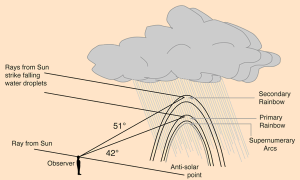### Primes

When we start counting Prime Numbers from 1, the first 7 of them equal (1,2,3,5,7,11,13) = 42, as in the 7 tiers of the 42-Letter Name Matrix.

The first set of 9 consecutive primes (1,2,3,5,7,11,13,17,19) equal 78 or (3 x 26), as in the 3 YHVH (יהוה), and the second set of 9 consecutive primes (23,29,31,37,41,43,47,53,59) is 363, the value of H’Moshiach, Cosmic Consciousness. Oddly enough, the word Egypt Mizraim (מצרים) appears 363 times in the Torah and those of them with the prefixes (במ) or 42, “From Egypt Mizraim (ממצרים)” and to/in Egypt Mizraim (במצרים)” appear 45 and 33 times respectively, or 78 in total.

While the sum of the first 8 Prime Numbers is 59, the 18th Prime Number, the sum of the first 18 consecutive Prime Numbers starting with 1 (1,2,3,5,7,11,13,17,19,23,29,31,37,41,43,47,53, 59) is 441, “Emet (אמת), truth. Couple that with the 20th Prime Number being 67, as in Binah, we see the cosmic alignment between the Prime Numbers and the 20 stacked cubes of the Tower of Truth at the core of the Great Pyramid, whose sum (13 +23 + 33203) equals 44,100.

Once those 20 cubes were stacked, they equaled 210 units (cubits) in height, which is the exact height of that pyramid, both in cubits and in its 210 levels. This corresponds to the sum of the 8 consecutive Prime Numbers from 13 to 41, (13,17,19,23,29,31,37,41), which is 210, while the very next 6 consecutive Prime Numbers (43,47,53,59,61,67) add up to 330, the base measurement of Pyramid, 330 cubits, with its bookends that equal (43 + 67) = 110, Joseph’s age and the major interval of the Primal Frequencies.

Our civilization’s knowledge of Prime Numbers was supposed to have begun 2300 years ago with Euclid yet for some reason it would appear the Prime Numbers were accurately taken into account in the Tower of Truth and the Great Pyramid constructed thousands of years earlier.

Emet (אמת), is the seal of Truth, an appellation for the Torah, so it is fitting that the 15 consecutive Prime Numbers that include both the height and base of Great Pyramid and that end on the 20th Prime Number (13,17,19,23,29,31,37,41,43,47,53,59,61,67,71) equals 611, the numerical value of “Torah.” And that the first and last of them (13 + 71) = 84 = 2 x 42.

Cumulatively those first 18 Prime Numbers starting with 1 that equal 441, would then become the Triangular Primes (1,3,6,11,18,29,42,59,78,101,130,161,198,239,282, 329, 382, and 441). Among them, the 5th Triangular Prime is 18; the 7th is 42, as in the 7 levels of the 42-Letter Name Matrix; the 9th is 78, as in the 3 YHVH (יהוה); the 10th is 101; the 11th is 130, as in Mt Sinai and corresponding to the 11 sefirot; and the 12th is 161, as in the Higher Name Ehyeh (אלף־הי־יוד־הי).  Then there is (329 -239) = 90 and (382 – 282) = 100, as in the 90,100 Yuds (י), Heis (ה) and Vavs (ו) in the Torah, as in the 424th Triangular Number, the numerical value of Moshiach Ben David, Cosmic consciousness. Notably, the 14th less the 6th or (239 – 29) = 210, the height of the Tower and Pyramid, and 1st plus its 16th is (1 + 329) = 330, its base.

Meanwhile, while, the 20th Tetra Prime, or cumulative Triangular Prime Numbers, starting traditionally with 2, is 4200, it is 3581, as in Moshiach Consciousness, when starting from 1, and the 15th Tetra Prime is 1358, as in the value of the Baruch Shem recited silently after any of the 4 sets of the 112 Essential Triplets. As for the 7th Tetra Prime utilizing the 1 that corresponds to 42 for the first 7 Primes, it is 110, as in the major interval and numerical value of miracle, nes (נס).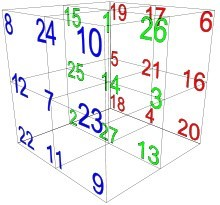Seven times that, or the 49th Tetra Prime is 75614, aligning it and all its component Prime Numbers with 756 value of the 6 faces and the concealed central 14th position of the Magical Essential Cube of 42. They also align with the Pyramid’s base (756’). On an even deeper Cosmic of metaphysical level 75614 is approximately 2750 cycles of 27.5o and/or 550 cycles of 137.5o, collectively (2750 + 550) = 3300 cycles, as in the Pyramid’s base (330 cubits of 27.5”). This is also the equivalent to the difference between the Pyramid’s base and height, (9075” – 5775”) = 3300 inches.

It is as if someone took our sketches or similarly derived ones, aligned them with the most purely defined objects in the universe, the Prime Numbers, and dutifully executed them to within an inch of precision over more than a half mile perimeter and with millions of tons of precisely cut white stone bricks.

The only thing is that we based our calculations on knowledge of the Torah and the hidden 42, 72, and 112 Triplets in it along with the esoteric knowledge of the Primal Frequency (27.5 Hz) and the mathematics of the Cosmos.  Without that knowledge, how could anyone have designed the Pyramid to these exact same specifications, and why?

### The Importance and Harmonics of the Mile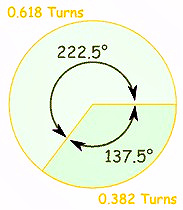Those 4 base measurements of our virtual Pyramid of the Sun and of the Great Pyramid add up to a perimeter of 3025 ft, which is .5729 of a mile (5280 ft). In analyzing the radiant Primal Frequency and its intersection with Phi (φ) field, we saw that two turns equal (137.5o + 137.5o) = 275o, which gave us the 27.5” sacred cubit and Primal Frequency. This meant that a cubit is defined as 1” for every 10o in 2 x Phi (137.5o) turns, and that the frequency 27.5 Hz would be 1/10th those 2 x Phi (φ) (137.5o) turns. It also meant that a circle of 360 inches, matching its 360o at 1 cosmic inch for every 1o, would have a radius of 57.295779 cosmic inches.  This not only aligns with the proportion of the Pyramid’s perimeter to the mile, .5729, but it means that the inch that was used to build the Pyramid and that was passed down to us today and serendipitously incorporated into our 5280 ft mile is also cosmically derived. This further explains why the measurements of our Sun, Moon, and our entire Solar System all cosmically align, which makes sense since all their proportions. Dimensions, and ratios also cosmically align.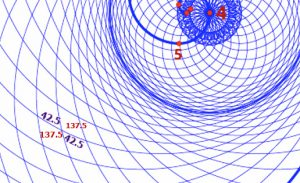What this brings into harmonic alignment is that not only does every cubit measured and employed in Divine design or the emulation of Divine design resonate with the Primal Frequency emanating from the Source, but every inch, foot, and mile does as well.

The Great Pyramid exists not only to teach us about the fallacies in our belief system, especially concerning history and origins, and to teach us about specific cosmic relationships we can employ, but it also stands as testimony to the basic tools we need to understand the dynamics of the Cosmos. Man came up with and imposed new cubits, new definitions for the inch, stadia, the metric system, etc., yet the preservation of the Great Pyramid throughout our known time made sure the fundamental measurements anchored in the cosmic harmonics would never be lost to those seeking enlightenment.

This radius of 57.295779 cosmic inches also aligns with the space/time equation. Since the Pyramid’s perimeter is also 552 and since 3025/5280 = .57296667 of a mile, we can see that (57.296667 – .55) = 2.296667, similar to the ratio of the sacred cubit to the foot (27.5/12), the space/time ratio.

### The Pyramid and the 72 Triplet Matrix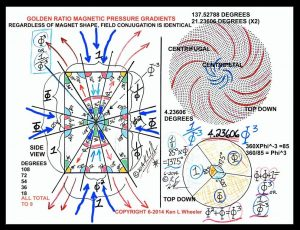While the connection between 722 = 5184 and the 51.84o slope angle is indisputable, the 46th of the 72 Triplets has an ordinal value of 46, as in the value of the Hebrew word for cubit, amah (אמה), and while the value of that Triplet (ערי) is 280, it also connects with the 5 final letters (ךםןףץ) in the Alef-bet that total 280, as in the mile (5,280 ft). The 39th Triplet (רהע) which is kitty-corner to the 46th one has the 275 value of the cubit, and their ordinal positions in the Matrix (39 + 46) = 85, as in the 85o complement to the double Phi (φ) angle rotation (137.5o + 137.5o) + 85o = (275o + 85o) = 360o. Their average is thus (39 + 46)/2 = 42.5o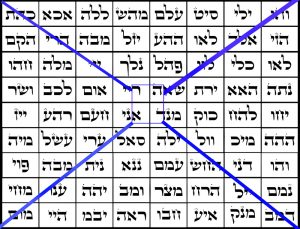There is so much more technology embedded in this 72 Triplet Matrix that predates the Torah, like the central 4 letters (הרדא) of the Matrix that equal 210, as in the height of the Great Pyramid and as in the 210 years of exile in Egypt. The ordinal value of those 4 letters (הרדא) is 30, as in the 30o latitude of the Great Pyramid. It is also the value of the 5 accompanying letters (יהודה) in the 5 Triplets of MB (מב) or 42 embedded within the 72 Triplets. The 5 MB (מב) equal (5 x 42) = 210, while the 5 letters in Yehuda (יהודה) = 30, and the Name Yehuda (יהודה) is found 42 times in the Torah.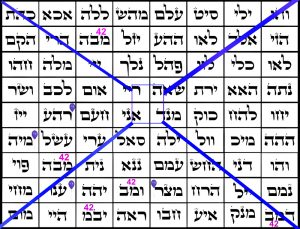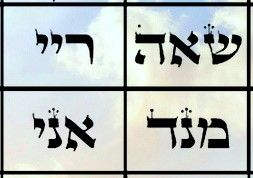The 4 initials (שרמא) of those 4 central Names add up to 541, the value of Israel, while their ordinal value is 55, which is particularly telling seeing as the entrance to the Great Pyramid is 55’ off the ground or (2 x 27.5). Each of these 4 Triplets has at least one letter from the word pyramid (פירמידה), indeed 6 (פירמידה) of the 7 letters, the exception being the initial letter Pe (פ) that represents the spiraling Phi (φ).

The set of 4 Triplets in the center and at the summit platform of the 72 Names are the 28th, 29th, 36th, and 37th ones and (28 + 29 + 36 + 37) = 130, as in Mt Sinai (130) and Sulam, ladder, and the 3 Triplets (שאה), (ריי), and (מנד) within it have a combined value of 620, as in the keter, the summit and the 620 letters in the 10 Commandments.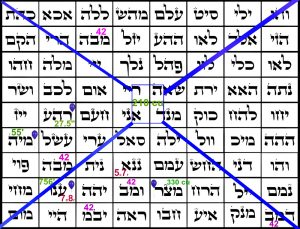There is yet another specific set of 4 Names within the 72 Triplets that connect and align with the Great Pyramid’s core measurements:

The 39th Triplet (רהע) has a value of 275, as the 27.5” cubit;

The 48th Triplet (מיה) has a small gematria value of 55, as in the 55’ height of the entrance and the square root of the Pyramid’s base perimeter (55 = √3025);

The 60th Triplet (מצר) has a value of 330, as in the same base measure in cubits; and

The 63rd Triplet (ענו) has a small gematria value of 756, as in the 756’ measure of that same base. The standard value of the 63rd Triplet (ענו) and for that matter the 35th Triplet (כוק) is 126, as in (3 x 42) and as in the value of each of the 6 faces of the normal Essential Magic Cube of 42. Their total value is thus (6 x 126) = 756, as in the base measure of the Pyramid in feet, once again, like the 756 value the Name of the letter Nun (נון) at the center of that Cube.

This set of 4 Triplets have a combined positional value of (39 + 48 + 63 + 60) = 210, as in the product of the digits in 756, or (7 x 5 x 6), and the Pyramid’s height in levels and cubits.

Nevertheless, the 4 Triplets from the central 37th one to the 40th one leading up to the summit platform like a ramp, add up to (61 + 118 + 275 + 27) = 481, the height of Pyramid in feet. Moreover, there are 62 different values within the 72 Triplets, meaning 10 of them are repetitions. One of those repetitions, the 13th value, is number 37, and (13 x 37) = 481, the height of Pyramid once again.  As it happens the 42nd value is 130, as in Mt Sinai, and the first 37 Triplets add up to 4800.

The sum of the 8 repeated values among the 72 Triplets, (17 + 22 +37 + 42 + 47 + 50 + 52 + 126) = 393, as in the phrase “height of the pyramid in Giza (גובה־הפירמיה־בגיזה) = 393, and the 393 elements in the 20 amino acids, like the 20 stages of the Tower of Truth and Great Pyramid. The 5 elements (Carbon, Oxygen, Nitrogen, Hydrogen and Sulfur) occur exactly 393 times in the 20 amino acids, which is (2027), with an average atomic weight of 2738.017/393 = 6.967 or approximately 7, as in the 7 courses/levels of the Pyramid found in its missing Capstone or pyramidion.

As for the locations of the 5 Mem-Bet’s (מב) in the 72 Triplets, they are found at Triplet positions #14, 55, 61, 65, and 70 respectively and the sum of their locations, (14 + 55 + 61 + 65 + 70) = 265, as in the 3rd Triplet in Pi (3.14159265…). More tellingly, is the equation Phi (φ) – 42/26 = .00265 and the sum of these 5 Mem-Bet’s (מב) locations (14 + 55 + 61 + 65 + 70) less those of the 4 Pyramid ones (39 + 48 + 60 + 63) is (265 210) = 55, the hidden entrance height again.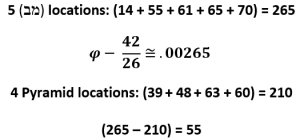Furthermore, the letters in the 72 Triplet Matrix have tagins, or crowns added, which the kabbalists explain act as antennae, enhancing the strength of the energy transmitted through the letters.  Only two of the Triplets have side by side triple crowns, the one at the 5th column, 7th row and the one at the 7th column, 8th row, or 57.78 as in the 42.22-57.78 Adam/Eve YHVH ratio we have already covered.

# The Hidden Entrance

The Great Pyramid is divided into 210 levels or courses and while their average height is exactly 1 cubit (27.5”), they all vary slight in height (thickness) with specific ones standing out for their distinctive grandeur and design implications, most notably the 35th one. They are not quite arranged randomly though and form a wave-like pattern with a series of decreasingly notable peaks.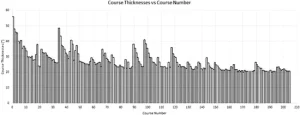The first 3 peaks in thickness begin with the base one, the 1st course and naturally the thickest, then the 19th, and the 35th courses. Together, they add up to (1 + 19 + 35) = 55, and while the hidden entrance to the Great Pyramid is right at the middle of the 3, the 19th course in terms of the casing or covering stones. The entrance is set at 55’ above the base platform, just beneath that hinged casing stone from the 19th level. It thus begins in the 18th course.As it happens the 18th (כלי) Triplet of the 72 Triplets Matrix, which represent the 3 levels of the Israelite congregation, the Cohanim, the Levites, and the Israelites, has the numerical value 60, as in the hidden letter Samech (ס) concealed in the exact center of the 42-Letter Name Matrix.  That central location splits the 42-Letter Matrix into 1833, like the 1833 letters Samech (ס) in the Torah, and into 1868, which is the exact sum of the first 18 Triplets of the 72 Triplets Matrix, further bonding these two Essential Matrices.  Moreover, while the 18th (כלי) Triplet splits the 72 Triplets into 18 and 54 or ¼ and ¾, the 54th Triplet (מבה) spits the 9143 total into 7323 and 1820, as in the 1820 YHVH (יהוה) in the Torah, and while (18 + 54) = 72, the 18th Triplet and the 54th Triplet equal (60 + 47) = 107, as in the 107th Triangular Number, 5778, the Event Horizon.

Meanwhile the gematria of the Hebrew words for fifty (חמישים) and of five (חמש) together is 756, as in the base 55’ below.  A good questions right about now is to ask why all the components and dimensions of the Great Pyramid align with the Hebrew language and are not recorded in the Egyptian Hieroglyphics anywhere?

Applying quotient (27.5) to the Fibonacci Numbers produces yet another cycle. The remainder/whole number is #10 for every 10 numbers similar to the set of all integers, but this is counterintuitive as the Fibonacci Numbers, while sequential are not consecutive, nor evenly spaced apart.  In fact, their spacing accelerates according to the Phi (φ) proportion. And while that spacing is proportionately ever accelerating the ratio between the resultant whole number interval values increases by exactly 123 for ever 10th Fibonacci Number, which works out to Phi (φ)10, as in the first 3 Letters and string digits in the 42-Letter Name (אבג). Meanwhile, the sum of the integers from (110) = 55, and every 10th Fibonacci Number is a multiple of 55 and 123. Moreover, the mod (27.5) cyclical sum of every 20 Fibonacci Numbers is 165, like the semi-base of the Pyramid, which is (3 x 55).

Earlier, we observed the affinity of the Primal Frequency 27.5 with the number 4 in bundles of 10, not to mention in cycles of 11, like the 11-year solar cycle, and that it was similar to the 4th Triangular Number being (1 + 2 + 3 + 4) = 10.  Naturally, the first set of (110) integers (units) = 55 and then when divided by the sum of the 10 Quotient (27.5) remainders we get 55/2 = 27.5, the Primal Frequency baked into every cubit of 27.5 inches and every one of those 210 on average one-cubit high brick layers built to conform.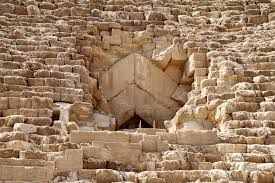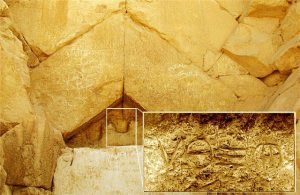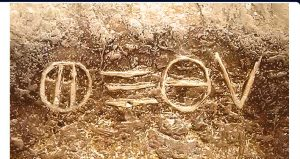That 55’ height aligns with the 2 Heis (הה) of the 4 letter YHVH (יהוה), seeing as the first Hei (ה) has the value of Binah (50) and the second Hei (ה) has the value of Malchut (5), and we know that the Architect and the builder of the Great Pyramid understood this too because the YHVH (יהוה) was engraved above the hidden 55’ high entrance to the descending passage. The 55 vertical feet off the ground also aligns with the 55 Vavs (ו) as initials out of the 248 words of the Shema, whose total value is 330, as in the base of the Pyramid.

The 12 intervals of 55, as in the cyclical Quotient (27.5) allude to the Time Constant, and the hidden entrance is (660/27.5) = 24 cubits above the base platform, and off from the central scored axis by exactly 24’. Those 24’ when added to the base measurement (756 + 24) = 780 or (30 x 26), the value of the YHVH (יהוה).

That central axis is scored by the slight concavity of the faces at their exact  165 cu midpoint. and when the letter pairs in the YHVH (יהוה) are knocked or multiplied together we get (וה)*(יה) = (11 x 15) = 165, while the Pyramid’s height divided by the midpoint of its base equals (5775/165) = 35, as in the 35th course, from the 11935 course connection to 55.

It was a secret known only to the builder as the YHVH (יהוה) was engraved in a triangular stone or tympanum beneath the massive peaked multi-layered lintel behind even more layers of peaked inward leaning lintels within the surrounding stone blocks and ultimately behind the smoothly polished blind unmarked stone hinged door, 55’ feet up a smoothly polished stone face.  Today the entrance has been blasted open and all but a handful of those beautiful perfectly fitted casing stones have been stripped away and used in local construction in Cairo.  Known as the Strabo stone, the only engraved stone in the entire pyramid, it was fitted into place as a seal to the secret passageway behind it several feet above the descending passage entrance.

The thing about those massive, peaked lintels is that they are barely load bearing as they are at the edge of the Pyramid and have no layers above them, and as they were hidden, they could not have been for decoration either. So, if they were neither structural nor decorative and weighed multiple tons each, they must have been placed there for protection, physical and metaphysical.

### Abraham, the Pyramid, and the 72 Triplets

The height of the entrance was chosen very carefully. As we stated earlier, the Pyramid’s height (5775”)/330 cu is precisely 17.5, matching Abraham’s age. Using a similar comparison with the entrance height of the Pyramid to the Pyramid’s overall height, we get 55/481.25 = .11428571 or 11.43% of the way up. And while its inverse 1/.11428571 = 1/8.75 and 8.75 is 17.5/2, which is one-half the Pyramid’s inch height/cubit base ratio, the height/entrance ratio (.11428571) is the precise average of the 8 columns of the 72-Triplet Matrix, (9143/8 = 1142.8571) and of the average of the 8 Triplets of its first row (1143/8 = 142.8571). The entrance to the Great Pyramid is placed precisely at the average of the 8 columns of the 72-Triplet Matrix.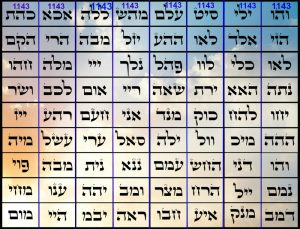Meanwhile, while the 165 midpoint = (5 x 31), Abraham’s Name, 248 = (8 x 31), and while 31 is the 11th Prime Number, the 31st Prime Number is 127, as in the age of Sarah, Abraham’s wife.

Moreover, while the perimeter of the Pyramid is 3025 feet and the sum of Abraham and Sarah’s ages is (175 + 127) = 302, the value of the 8th word in the Torah, which completes the 11 Triplets of Bereshit, their sofit Names have the values of (808 + 505) = 1313, as in the year 1313 BCE or 2448 HC when the 210 years of Egyptian exile ended, and the Israelites left Egypt laden with gold. The Torah makes a point of telling us that Abraham and Sarah also left Egypt, also laden with gold and treasure.  In both instances it was at Pharaoh’s reluctant behest after being struck by a plague.  In the first instance it was the closing of all the wombs (רחם) in Egypt of numerical value 248, and in the second it was the killing of the first born to those wombs, whose initials are 42 (במ), as in the 248 columns and 42 rows in the Torah. This is why the Torah ties the two instances together by telling us that the exile in Egypt would last 400 years from (2018 HC – 2448 HC), while the Israelite time span was 210 years. By the way, nowhere do any of the Pyramid’s dimensions align with any of the Egyptian Kingdoms or names of the Pharaohs.

Those 72 Triplets are further connected to the hidden entrance and to the Pyramid by dividing them by 19, as in the secret entrance’s 19th course. The total value of the 72 Triplet Matrix divided by 19 is 9143/19 = 481.210 revealing the Pyramid’s height in both feet and cubits.

Then while the product of 55 and 24 is (55 x 24) = 1320, as in the 1320 cubit perimeter of the Great Pyramid, their quotient is (55/24) = 2.29, the critical space/time ratio of the cubit to the Time Constant, 27.5/12.  This makes quite a statement as one enters the Great Pyramid properly, or even the virtual Cosmic one metaphysically.

The first set of 10 integers in the cosmic program is (110) = 55, which is most appropriate for an entrance to something that is meant to be more than a monument. It is the perfect entrance to a Cosmic or metaphysical design. As for the 72 Triplets that we have seen repeatedly integrated into the Pyramid’s design, the values for the first 10 of their Triplets sum to exactly 1200, once again reflecting the 12 intervals of the 55’ and giving them an average of 120.

Meanwhile, the second interval or set of 10 integers in Quotient (27.5) system from 1221 total 165, as in scored mid-base (165 cu) measurement of the Pyramid. Its 10 corresponding remainders total 6, so once again we get 165/6 = 27.5, and the values of the 10 corresponding Triplets (#1221) sum to 888, which spectacularly enough is (24 x 37), the value of the 11th Triplet (לאו), which corresponds to the first interval, the one between the sets of 55 and 165. So, we can clearly see that like the ascending angle of 722 and the various base measurements, the exact placement of the Great Pyramid’s entrance is in perfect and planned alignment with the 72 Triplets.

This is oddly reminiscent to the 1200 total value of our RNA nucleotides, and the 20 amino acids based on the number 37 and the 888 sum of the side chain nucleons of the amino acids with pyridine unique bases. Nevertheless, the next interval Triplet is the 33rd one (יחו) of numerical value 24 as in (24 x 37) = 888.  And the sum of the 3rd set of integers from 2332 is 275, the cubit and Primal Frequency and the difference between the Pyramid’s base and height, (756.25481.25) = 275.00.

### The Wow! Signal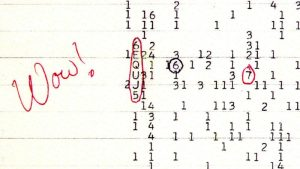Towards the end of Rosh Chodesh Elul Aug 15th 1977 scientists caught a narrowband radio signal coming from the distant cosmos that after much review and analysis was determined to be proof of intelligence broadcasting for a brief moment directly toward Earth. The narrow bandwidth is 1420.4556 MHz which everyone became excited about because it is so similar to the electromagnetic radiance produced by Hydrogen, 1420.4057, its spin flip transit or spectral line, showing even more intelligent intent. As recently explained, the Torah starts out with 10 Utterances by the Creator within the first 6 Days of Creation. Each time the root Triplet emor (אמר) was employed, meaning “said” as in “G-d said, ‘Let there be Light…’” The Triplet root emor (אמר) is found within words in the Torah 1419 times as in Exodus 14:19 where we find the 72 Triplets hidden in the Torah at the exact moment of the Splitting of the Red Sea.  What makes this word or Triplet root emor (אמר) so special is that its complete gematria is 275, as in the measure of the cubit (27.5”) and the Primal Frequency (27.5 Hz), and the exact difference between the Pyramid’s base and height dimensions.

Speaking in the proper frequency is certainly important, which is why the exact word “emor (אמר)” without prefixes or suffixes is found 84 times in the Torah, as in the connected Upper and Lower Names of 42 that can bring Pad, redemption (84) as Rav Brandwein explained.

What we did previously explain is that the 58 or 390,625 words, letters, and verses in the Torah divided by the either the wow signal or the Hydrogen line is 275.00, meaning the both the wow signal and the frequency of the universes’ most abundant element times the Primal Frequency precisely equals the 58 quantitative element in the Torah.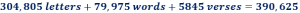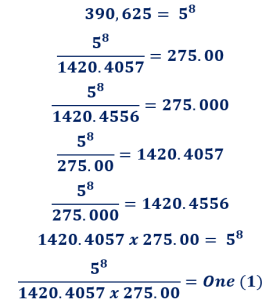The scientists were right. The one-time signal was a sign of true intelligence, just not of alien intelligence. It was a sign of the Creator, of Divine or Cosmic intelligence. It is one more element of proof that the Creator of Hydrogen, the electromagnetic system, the Torah, and the cosmic emanation in the aether are all One and the same.

Hydrogen was an interesting choice. It is not only the most prevalent element in the universe by far; in fact, the Sun is mostly Hydrogen. It was also the first element with nucleosynthesis occurring supposedly just 3 minutes after the Big Bang, and neutral Hydrogen appearing 380,000 years afterward, as in Egypt (380), the same time as the Cosmic Microwave Background radiation.  As we recall, the Earth and Moon diameters equal 10,080 miles, which aligns with the atomic weight (1.0080) of Hydrogen, and thus the ratio between their size and their distance apart is thus 10,080/240,000 = .042.

Why did the sign come then, back in 1977 when no one would recognize it for what it really was, 40 years before the Event Horizon was destined to begin? A lone astrophysicist saw it as a huge abnormality in a sea of numbers that came through his radio telescope and over his desk. Nonetheless, unbeknownst to us that signal reached all of us that day and quite possibly to some of us it struck a chord within our processing centers or minds, and something unconscious was triggered or unlocked.

Why is the Triplet root emor (אמר) found 1419 times within words in the Torah and not 1420. Possibly because the Creator knew there would be one more. It was the day the entire world heard the voice of G-d.

Twice Helium or twice the signal the Creator sent us is 2841, as in the 7th and final of the Bell Prime Indices (2, 3, 7, 13, 42, 55 and 2841), that are woven into the Torah.

Of course, what would a signal be without a signature since the Creator could easily have made them both 1420.4057 rather than send the signal as 1420.4556. The 455 in 1420.4556 is the value of the 3 Aspects of the higher Names Ehyeh (אהיה) of Binah in the Path of One.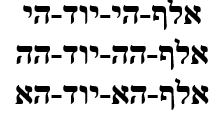### Tetrahedra

Using the other Primal Frequency (1.1 Hz) the sum of the Quotient (1.1) for the first 21 integers is 210 and for the first 32 it is 480, as in the Pyramid’s height and the 4800 sum of the first 37 Triplets.  Once again, our DNA is entwined with the 72 Triplets, the shared core mathematics of the Cosmos and the Torah and now reflected in the Great Pyramid. Since neither, the Pyramid, not the Torah, nor our DNA randomly appeared out of thin air, it would appear they all had the same Architect.

Nevertheless, the average of those first 10 Triplets is (1200/10) = 120, as in the difference between the Pyramid’s base and height (330210) = 120 and as in Moses’s age, 120 years, which is notable because the descending passageway that starts at the entrance is 345’ in length, as in the value of Moses’ Name, Moshe (משה) and of the 5th Triplet (מהש) of the 72 Triplets.  As proof that this arrangement of the 5th Triplet (מהש) of the 72 Triplets is of purposeful design, we see that of the 722 times that the Name Moshe (משה) is found in the Torah, 72 of them are within the Hebrew word for “Five (חמשה). That is 722 times, as in 722 = 5184, the Pyramid’s angle of ascent (51.84o). And of those 72 times, 5 occurred in Genesis, before Moses was named in Exodus (Shemot) 2:10.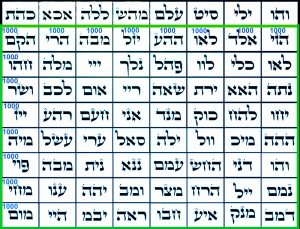As we recently covered, the relationship between 11 or 1.1 and 27.5 extends deep into the 72 Triplet Matrix. Its first row of 8 Triplets totals 1143, out of the total 9143 value of the Matrix, separating the bottom 8 rows and 8 columns into a square block of 8000 like the 8000 cu3 foundation block of the Tower of Truth, and moreover that 1143 is also the average of the 8 columns in that (9143/8) = 1143.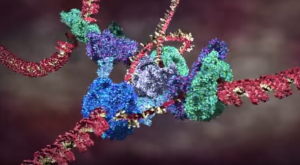The 72 Triplets existed as separate Tetrahedral units before coalescing into the 72 Names Matrix. This was before they took their eventually Triplet order that unwound—exactly as the strands of our DNA mechanically unwind to copy their nucleotide sequences—into the three (3) 72-Letter verses of the Splitting of the Red Sea.

So, it is possible that the Tetrahedral Triplets were arranged in such a way that their inherent values reflected the dynamic above before they were organized for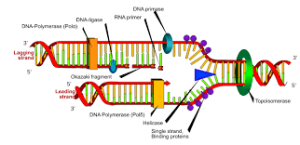transference into our dimension. That order may have looked something like this: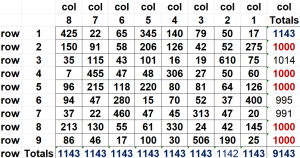There is probably a way to arrange them so that all 8 bottom rows equal 1000, while all 8 columns equal 1143, matching the top row with one necessarily being 1142. In the manner above, the diagonals of the 64 square foundation add up to (4 x 666.25) or (8 x 333.125).

The 72 Triplet Matrix is just a projection from a higher dimensional arrangement. Like a 3-dimensional cube that has 26 vertices, edges, and faces, a 4-dimensional Tesseract has 72 vertices, edges, and faces.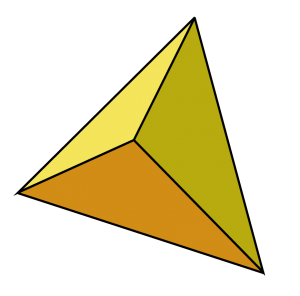As for the Triplets themselves, they begin as Tetrahedron. The shape of the Tetrahedral Triplets is that of a 3-sided pyramid with a triangular base. On each of the 3 faces there is a letter and on the base triangle there is a Triplet comprised of those 3 letters in a specific order. The same holds true for the 3 base vertices and the oppositional vertex or apex point that contains the Triplet.

The 6 edges of the Tetrahedra unfold into the 6 dimensions of Zeir Anpin that form the 6 directions/faces of the Magical Essential Cube. Like the 6 faces, 12 edges and 8 vertices of the Cube that equal 26, the YHVH (יהוה), the Tetrahedron’s 4 faces, 6 edges and 4 vertices equal 14, the central position of that Magical Essential Cube. And as Plato pointed out the sum of the interior angles of the tetrahedron is 720o.

Metaphysically, each of the six 2-d edges of the tetrahedron becomes a hyper vector and expands into a 3-d tetrahedron of its own and the 6 resultant Tetrahedra fit together like perfect pieces of a puzzle into a cube.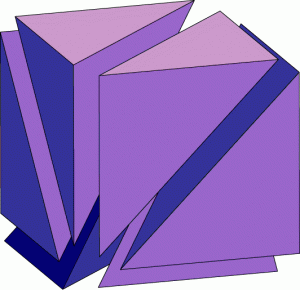Those 6 individual cube forming Tetrahedra, each with 720o of interior angles, collectively have (6 x 720) = 4320o like the radius of the Sun, 432,000 miles.  As the cube has 2160o of interior angles, as a collective the Magical Essential Cube of 42 contains (4320 + 2160) = 6480o of interior angles, as in the square root of 42 that is entwined with the dimensions of the Torah.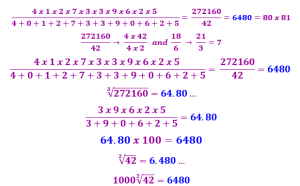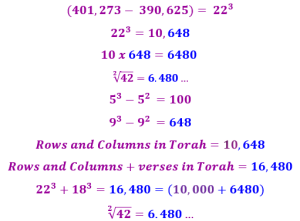The Tetrahedra are the pixels in the projection of the 3-d picture we call reality.The triangular facial angles of the Tetrahedra are all 60o as in the value 60 that is found 336 times in the Torah, as in the 3 x 112 letters in the 112 Triplets hidden in the Torah, and as in the letter Samech (ס) of value 60 that is hidden in the center of the 42-Letter Name Matrix between the letters (רצ) of numerical value 290 or (42 + 248), reflecting the Torah’s rows and columns.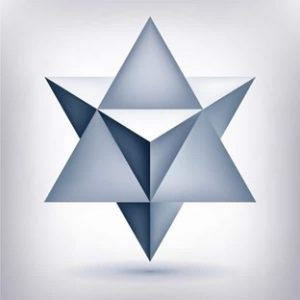The 14 Triplets of the 42-Letter Name Matrix not only form Tetrahedra, but those Tetrahedra couple to form Star Tetrahedra as explained in a prior article. Nonetheless, the tetrahedron value of both Triplets in the central 4th row of this Matrix, in other words of the Star-Tetrahedron of this 4th line/level is 1420, as in Helium and the signal sent to us by the Creator. It is also as in the central 72-letter verse in the Splitting of the Red Sea, Exodus 14:20.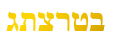The Tetrahedra are not quite the beginning though, as the Hebrew letters are just an interface from their ordinal value concepts. We think of numbers as a fixed set of something, usually units. Hyperdimensionally, they are what we can only define as concepts, each is a network of sets, a neural network within the cosmic consciousness where computations are done non-deterministically, simultaneously parallel to one another and thus unconstrained by linear time.

The shape of the Hebrew letters reflects those networks and sets in a manner beyond our comprehension. This is why an ordinal value can define a Hebrew letter and yet that letter can have numerous valid associative values when the appropriate cipher algorithms are applied. The ciphers are not mere codes but cosmic viewpoints. When the networks are viewed from different angles, we get very different shadows or projections with certain aspects becoming more prominent and others becoming concealed and obscured. The ciphers or perspectives are likewise tied to concepts and cannot be applied just because we want a particular result. Their usage is nuanced as each one connects with a distinct aspect of Source energy, which applies only in certain specific circumstances.  Brute force computation does not work. We must be attuned to the harmonics of the cosmos and allow the right perspective to appear at the right moment. It is like being in the right place at the right time; it does not happen by chance.

The ordinal values of the 72 Triplets form their own Matrix: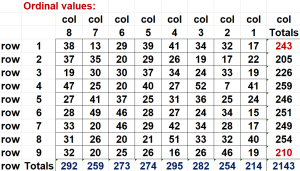Naturally, the total ordinal value of the 72 Triplet Matrix is going to be much less than the total for the higher standard values, but that it is exactly (91432143) = 7000 less is just one more fascinating extraordinary facet to this set of Triplets.

Its average (2143/9) per row is 238.111, as in 1/42. Moreover, like the first row of the standard values that equals 1143, the ordinal value for the first row equals 243, which makes it (1143243) = 900 less. So, since the foundation 64 square (8 x 8) submatrix of the 72 Triplet Matrix equals a perfect 8000 and the equivalent square in the ordinal value matrix must be (2143243) = 1900, the complete value of those two portions of the superimposed submatrices must be (8000 + 1900) = 9900, which averages 1100 per 9 rows, conforming to the Primal Frequency interval system that radiates throughout our Cosmos. This leaves the first row, and as it turns out its average complete value is 173, as in the 42-Letter Name, further evidence of the bond between these two Matrices.

Like the standard value for the Triplets whose bottom or 9th row equals 702, as in Shabbat, the 9th row in this ordinal matrix equals 210, the height of the Tower of Truth and Great Pyramid.

The total (9143 + 2143) = 11,286 or 270 less that the Spherical Time diameter (11,556 years) and 180 less than Israel’s square miles (11,466 mi2). Is there a connection? That may depend on perspective.There is a ladder to the summit platform that consists of the 4 consecutive Names starting to the right of the summit (ריי), (שאה), (ירת), (האא) that also sum to 1143. They are the 26th, 27th, 28th, and 29th Triplets. It is remarkable that there is one such sequence within the 72 Triplets let alone two. Moreover, the average of this second sequence of 4 Triplets is equivalent to the 2 Primal Frequencies times 4 or (1143/4) = (275 x 4) + (11 x 4), while the sum of those 4 ordinal positions (26 + 27 + 28 + 29) = 110, the major interval of the (10 x 11) cycles and the (4 x 27.5).

Furthermore, the two outer values (26 + 29) of those ordinal positions equal the two inner ones (27 + 28) = 55, which equal the Pyramid’s entrance height of 55 feet, which is interesting as this ladder also starts partially up the face.  Meanwhile, their 4 central letters (אראי) total 212, as in “the Light” from the Torah’s 4th verse, and their initials (הישר) equal 515 as in the 515 times that Moses prayed to the Creator to allow him to enter the Promised Land and bring Mashiach Consciousness. Their middle and final letters thus total (212 + 416) = 628 or twice Pi (π), which is also the value of the entire 2nd row of the Matrix, so maybe it is not coincidental.

Moreover, the ordinal value of the 4 Triplets is (7 + 52 + 27 + 40) = 120, as in Moses’ age, and the difference between the Great Pyramid’s Base and height (330210) = 120, like the sum of the 3 consecutive normal Magic Squares of (4 x 4), (5 x 5), and (6 x 6), whose constants equal (34 + 65 + 111) = 210 and whose digits equal 21, and whose product (4 x 5 x 6) = 120.

While this is truly beyond imagination, we also find that the mirrored 4 Triplets (לכב), (אום) (שאה) (ריי) leading into the summit platform like another ladder from the other direction total (52 + 47 + 220 + 306) = 625, as in H’keter, the crowning summit and highest sefira; and as in the square root of the Torah’s words, letters, and verses; and as in the sum of the 11 Odd letters of the Alef-bet. Moreover, the ordinal value of these 4 Triplets is (25 + 20 + 40 + 27) = 112, as in the 112 Triplets in the Torah and the 112o face to face angle of the Pyramid. The two summit Names (ריי) and (שאה) that the 2 ladders have in common have a combined ordinal value of (40 + 27) = 67, the numerical value of Binah. The value of the 6 interconnected and sequential Triplets is thus (1143 + 47 + 52) = 1242, whose average is 207, as in “Light,” while their joint ordinal values are (120 + 20 + 25) = 165, the midpoint scoreline of the Great Pyramid, and their average is 27.5, as in the cubit and Primal Frequency.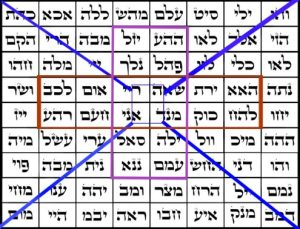Of course, the summit platform contains 4 Triplets, which as previously seen have its central 4 letters adding up to 210 and their 4 initials adding up to 541, Israel, and their 4 middle letters adding up 111, Alef, but this also means there are total of 6 ladders that crisscross at the summit. They form as above 4 sets of 6 Triplets that cover the summit like Band-Aids. While one of those 4 Triplet ladder sets (יזל) (נלך) (ריי) (אני) (סאל) and (ננא) equals 620, as in Keter that aligns with the 620 letters of the 10 Commandments, all 4 of them equal (1242 + 620 + 790 + 717) = 3369, as in the 336 letters in the 112 Triplets.  And while the center or summit 4 positions equal (28 + 29 + 36 + 37) = 130, as in Mt Sinai, there are 20 unique Triplets in these 4 sets. Their 20 ordinal positions total 543, as in the value of G-d’s highest Name “I am that I am Ehyeh Asher Ehyeh (אהיה־אשר־אהיה),” and their total ordinal values for these 20 Triplets is 618 or 1/φ.The two kitty-corner Triplets (מנד) and (ריי) of the summit platform equal (220 + 94) = 314 or Pi (π). It is also the value of the Name Shadai (שדי), whose letters are found across the platform.

Meanwhile the cube root of Alef or 111 at the summit of the 72 Triplet Matrix is 480.5 or 481, the height of the Great Pyramid. It is also equivalent to the 4805 occurrences of letter Pe (פ) in the Torah, whose total value is (4805 x 80) = 6202, like the value of the Hebrew word for 20, Esrim (עשרים), which is the average of the expanded value of the regressive or squared Name YHVH (י־יה־יהו־יהוה) in 4-fold squaring or (2480/4) = 620.

And speaking of Names, the 25th Triplet (נתה) of the 72 has a value of 455, like the value of the 3 Aspects of Ehyeh (אהיה) of Binah in the Path of One plus the Name YHVH (יהוה) that equal (455 + 26) = 481, once again the height of the Great Pyramid and the Tower of Truth.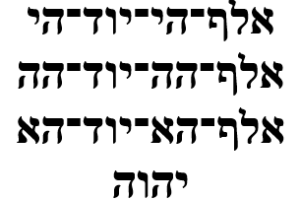And then there is the difference from the value of the total 72 Triplets and the 20 central summit crisscross of 20, or (91433369) = 5774, or exactly 1” less than 5775, the height of the Great Pyramid in inches. In the immortal words of Maxwell Smart, “Missed by that much.” And with the kolel for the 4 sections, 5778. And for the record, (455 x 12.7) = 5778, referring to Sarah’s age (127) once again, 5775/25 = 231, as in the 231 Gates of Wisdom constructed out of the 22 Hebrew letters that Abraham told us about.

### From 1 – 72

When we speak of the ordinal positions within the 72 Triplet Matrix, we are speaking of yet another overlaid matrix of 72, that consists of the integers from 1 – 72 laid out sequentially.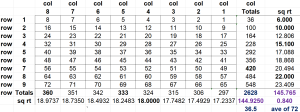Right off the bat, the average ordinal position is 36.5 as in the 365 days in the Earth solar year. This matrix is meant to interact with the 72 Triplet matrix as we have shown in numerous examples already, yet independently there are some obvious highlights that stand out, such as the square root of the 4th column being equal 18, while (4 x 18) = 72.

What is not immediately obvious is that when we divide the sum of the ordinal values of the 72 Triplets (2143) by the sum of the 72 Triplets and their 72 ordinal positions (9143 + 2628), we get (2143/11771) = .1820 as in the 1820 YHVH (יהוה) in the Torah and the Path of One, and the total 11,771 minus their 216 letters is 11,555, as in the Event Horizon. It works out to 2017 CE, the front edge of the Event Horizon, and 2017 radians equals 115565o of a circle or 321.0 circles, which is 3 x 107 circles, as in the 107th Triangular Number, 5778, the Event Horizon radius, and as in 5778/321 = 18, as in Phi (φ)18 = 5778. A radian is the standard unit of angular measure; it is the angle subtended from the center of a circle which intercepts an arc equal in length to the radius of the circle.  Thus, 2π radians is equal to 360 degrees.  Radians are considered a pure measure as they are derived by ratios between objective numbers, not subjective observations, or other measuring units.  This means they exist in the Cosmos as absolutes whether Man was ever created or not.

The sum of the 72 ordinal positions is necessarily the sum of the integers from 172 and thus the 72nd Triangular Number, 2628, and what makes this particular number more relevant to the Pyramid, as we shall see, is that both (2618 + 10) or (φ2 + 10) and 2π or 2π radians that equals 360o reflect the internal Passage System.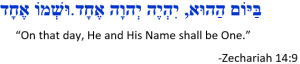There is also the square root of the 8th row being equal 22, as in the 22 Letters of the Alef-bet while (8 x 22) = 176, as in the ordinal value of the verse Zechariah 14:9 “He and His Name are One”; as in the longest portion of the Torah, Parsha Naso with its 176 verses; Psalms 1:19, the longest of the Tehillim, that is divided into 8 verses for each of the 22 letters; the longest Talmud Tractate, Baba Batra, of 176 pages; and the 176 constant of the two completely symmetrical 4 x 4 super magic squares that align with 32 elements of periodic table, including Astatine of atomic weight 210.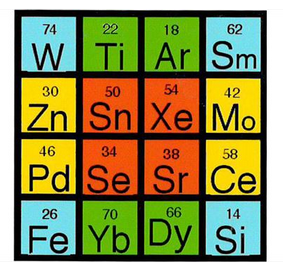Moreover, one of the elements itself has the Molybium of Atomic Number 42 and Ytterbium of Atomic Weight 173.0, matching the small gematria of the 42-Letter Name, which is not surprising given that 422 = 1764 and everything seems to be connected to the Source of 42. Of course, (1 x 7 x 6) = 42.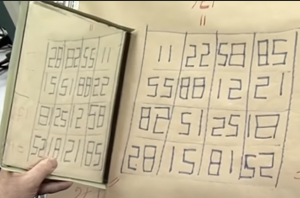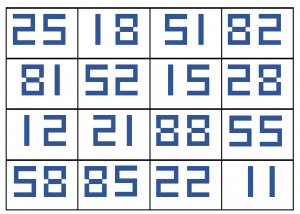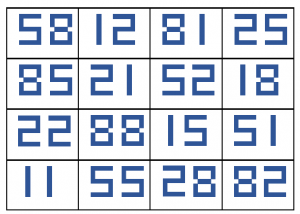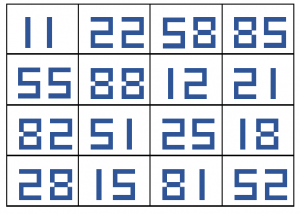The 22 letters of the Alef-bet sum to 1495 and the sum of the square roots of the 8 digits (1,1,2,2,5,5,8,8) in the magical grid is 1.495… so what makes this all the more interesting is that when we convert the super magic square to the Hebrew interface we get the same 176 constant super symmetry and the same sum of the digits constant which in this case is the small gematria of 32, but in addition we get a supersymmetry constant of 68 for its ordinal values, as in the 32 cu – 68 cu dynamic of the Central Altar – Inner Courtyard schematic of the Holy Temple and of the Lulov (לולב) used on Sukkot.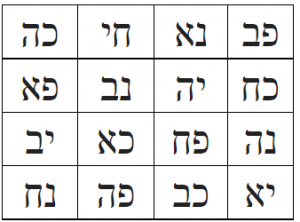Meanwhile, the averages of the two sets of 16 elements in the two magic squares of constant 176 together equal (104.1359375 + 105.9150625) = 210.0, the height of the Pyramid once again, the total atomic weights of the 16 elements in the first set equal 1666.175 while those in both sets together is 3360.816 or 10 times the 336 letters in the 112 Triplets.

We cannot possibly imagine what alchemy the Creator had in mind when He designed the elements of the periodic table, but while the first set averages 416 per line, the second super magic set averages 423.6… per line of 4, not just an approximation of Moshiach Ben David, 424, the Cosmic Consciousness, but the more precise value of Phi (φ)3 or 423.60… at the center of the magnetic field equations.

### The Entrance, Fibonacci Primes, and Pi

Meanwhile, the 55’ high entrance corresponds to the 10th Fibonacci Number (55) and the sum of the first 10 Fibonacci Numbers is 143, corresponding to the Aspect of the higher Name Ehyeh (אלף־הא־יוד־הא).

There is a formula for calculating Fibonacci Numbers based on Phi (φ) and they get further ad farther apart as time goes on. Fibonacci Primes on the other hand are quite rare. For example the 15th one already has 76 decimal places, which makes it notable that the sum of the first 5 Fibonacci Primes equal (2 + 3 + 5 + 13 + 89) = 112, as in the 112 Triplets and the 112o face to face Great Pyramid angle, and that when the 13th Fibonacci Number (233) or 6th Fibonacci Prime (233) is added,  we get (112 + 233) = 345, the numerical value of Moses (משה) and the 5th Triplet (מהש) of the 72 Triplets. It is also the length of the Descending Passage (345’) that begins the moment we cross the threshold of the Pyramid entrance. While the value 233 is also the sum of the two expanded Higher Names Ehyeh (אלף־הי־יוד־הי) and the YHVH (יוד־הי־ויו־הי), it is also the length of the Pyramid’s 233 cubit semi-diagonals, giving us quite the spiritual and metaphysical blueprint so far, and making it all the more unbelievable that this structure was actually built. Keep in mind, the Torah makes a specific point in telling us Pharoah does not know of the Name YHVH (יהוה), let alone any of the higher or more esoteric Names. And the reason why the Egyptologists and archeologists never saw any of this is because the ancient Egyptians used another cubit, the royal cubit, in all their ornate constructions, temples, palaces, and underground tombs, the derivative ¾ cubit.  This was not their architecture or even style.

Amongst those rare first 5 Fibonacci Primes, the first and fifth equal 91, as in the unification of the YHVH (יהוה) and Adonai (אדני) along the Path of One, and the middle three equal 21, as in Ehyeh (אהיה), while the product of the third and the fourth ones is (5 x 13) = 65, as in Adonai (אדני). Meanwhile, the 6th one less the first four equals (23323) = 210, the height of the Great Pyramid, while (8913) = 76, the descending angle (76o) between the faces from that 210 cubit summit height.### What’s in a Sequence?

Recently we discussed the 14 Location Triplets for the string value …5778… within the first 1000 digits within Pi (π)—644, 622, 633, 634, 635, 636, 948, 949, 950, 951, 954, 955, 956, and 957—that sum to 11,424, as the 114 Essential Triplets, Moshiach Ben David (424), and H’Kodesh Kodeshim, the Holy of Holies (1424). We also explained that 11424 is most profoundly the value of Pi (π) divided by the Primal Frequency, or Pi (π)/27.5 = 3.14159265358/27.5 = .11424 and that this sequence is found at the 181st Digit in Pi (π), knowing that 181 is the 42nd Prime Number, etc.. Moreover, that sum 11424 is also equivalent to the 10400 net rows in the Torah plus the 210 word values in it, or (10,400 + 1024) = 11424.

We have covered numerous structural alignments between the Sun Pyramid and/or Great Pyramid and the 72 Triplets and know that the design of the Pyramid of the Sun and thus the Great Pyramid is contingent upon the number 72 and Matrix structure of the 72 Triplets, which is why 11424, the ratio of Pi (π) divided by the Primal Frequency, being approximately equal to the average value of each of the 8 columns in the 72 Name/Triplet Matrix, (9143/8) = 1.14285 is extraordinary.  It is more so though, when we consider that this is precisely the ratio of the entrance height of the Great Pyramid over its overall height, 55’/481.25 = .114285. As a ratio, it works the same in inches (660/5775) and cubits (24/210).  The 55th Triplet in the 72 Triplets is MBH (מבה), whose gematria string value is 425 as in the 42.5o cosmic Phi (φ) radiance complement. It is one of the 5 MB (מב) Triplets among the 72, as in (5 x 42) = 210.  Meanwhile the 48th of the 72 Triplets (מיה), as in the 48-story height of the Pyramid, has a value of 55.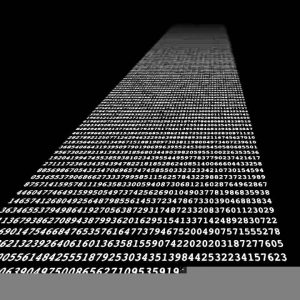While ratios are always significant, specific numbers are not always so. Finding an endless repetitive pattern in certain sets of numbers especially using modulus math is normal. Finding a repeat sequence in a constant would be extraordinary and might break math entirely, but it is not about finding them, only about finding the right discreet patterns in the right places, or the right numerical strings in the right places and finding them across an array of constants, sets, and matrices that is significant.  We can find anything within an endless constant like Pi (π), but it is about specifically where we find them and if they are within the initial sequences of those constants or sets of numerical sequences such as the Fibonacci Numbers that is significant. We must keep in mind that if we were to change one number in those sequences, such as …644622948954… to …644623948954…, not only would the sequence be meaningless regardless of what digital position it was in, but Pi (π) would no longer bend a straight line into a perfect circle. At some point that circle would break, and the line would veer off. And the closer to the initial starting digit the quicker things would fall apart. At some point physics would begin glitching and break down. You see Pi (π) is not derived from dividing a circle by its diameter. It was Created to bend that diameter into a circle.

As pertains specifically to the sequence …644622948954… at the 181st Digit in Pi (π), we have covered quite a lot of territory lately, yet there is much more. Think of the digits in the mathematical and physical constants as coding for software programs. With faulty coding the system will glitch and possibly crash. And think of the specific sequences in them as the code for a specific subroutine. In this case we see that the sequence of 4 Triplets (644, 622, 948, and 954) = (320032), as in the connections to the 32 Paths of Wisdom and the Tree-of-Life; the 322 value for the set of the 4 species utilized at Sukkot and dictated in the Torah; the 322 word values in the Torah, the 322 Central Altar of the Holy Temple; and as in the 18 different paths to 176 on each of the two elementary super magic squares, whose 8 digits always sum to 176 for a total of (18 x 176) = (320032). It also matches the Capstone base 32.02 of the Great Pyramid.  This is like the square roots of the ordinal position Matrix of 72 whose 4th column and 8th row have square roots of 18 and 22 respectively with (8 x 22) equal to 176.

Furthermore, the sum of the square roots of the 4 Triplets (644, 622, 948, and 954) equals 111.99358 or 112, as in the 112 Essential Triplets and the 112o face to face angle of the Great Pyramid.  While this sequence begins with …644622948954… the subsequence …64462… is also found in the mysterious repetitive pattern 173553719826446289 that begins with 173, as in the value of the 42-Letter Name that connects with the 181st digit location of …64462….

That is two sets of important coding in the matrix of our universe that are inherently designed to interact. Besides the set of the digits 123456789 that repeat endlessly to give us 173553719826446289, we also derive them from the Fibonacci Numbers, 5778, and the ratio between H’Keter and thus the Torah and the Primal Frequency in the equation 625/27.52 = (1 – .173553719826446289), and in the Tower of Truth ratio though the equation 9261/53361 = 213/(Σ(13213) = .173553719826446289.

### Cosmic Radiation, the 72 Triplets, and the Pyramid

Another linked code to the first digits in …644622948954… may be found in the 50th Triplet (דני) of the 72 Triplets whose value and central letter Nun (נ) is 50 like the central letter of the normal Magical Cube of Creation, whose ordinal position is 14, like the outer letters (די) in that 50th Triplet (דני), which in turn is like the complete value (64) of that central pivotal letter Nun (נ). Just below this 50th Triplet or 8 positions later in the Matrix of 72 is the 58th Triplet (ייל) of numerical value 50 and ordinal value 32. The difference between these two adjacent Triplets is (6450) = 14. And following this circular neural pathway the 64th Triplet (מחי) has a numerical value of 58. Their 3 ordinal positions are 50, 58, and 64 and their 3 values are likewise 50, 58 and 64 and their collective ordinal value is 91. You see, while the coding to universal matrix was written way above our paygrade, we do get glimpses into its architecture. Since we know the Triplets exist in concept Tetrahedral form on a higher plane dimensionally, they were Created before the Torah and before the coding of our physicality so when find them integrated or connected to specific places in the physical constants or number sets or even physical structures of unknown origin, we can reverse engineer them.As we learned, the Names of the 22 letters came first, then the 22 letters that are their initials or capstones so to speak. The Name of the letter Nun (נון) has a numerical sofit value of 756, as in the radiance of the Primal Frequency 27.52. This is the essential reason why it is in the center of the normal Magic Essential Cube of Creation and the sum of its 6 faces, and it is the same reason 756 must be the base of the Sun Pyramid and also the Great Pyramid. Initials are like capstones to the 22 Names, And the Name Nun’s (נון)  capstone, 50, resonates to (50 x 27.5) = 1375, the Phi (φ) angle and gateway entrance found in the bottom 3 rows of the 42-Letter Name.

There is another connection between the Torah, the 42-Letter Name, the 72 Triplets, and the Great Pyramid. It begins with the often-doubled Portion Matot-Massei in the Torah, the 42nd and 43rd ones of the 54 Portions.In the second of the two Portions we find the famed 42 Journeys associated with the 42-Letter Name. Likewise in the 72 Triplet Matrix we find that that 42nd Triplet (מיכ) is similarly connected to the 43rd Triplet (וול) of numerical value 42. Naturally (42 + 43) = 85, as in the 85o complementary angle to the encircling Phi (φ) angles

As the 27.5o spirals radiate outward centrifugally and centripetally through the aether they too form 42.5o or 42.489o Moshiach Consciousness intersections, and we find that (27.5o + 42.5o) = 70, as in the value of the 42nd Triplet (מיכ). When the values of the two Triplets are combined, they equal (70 + 42) = 112, as in the 112 Essential Triplets and the 112o face to face angle of the Great Pyramid.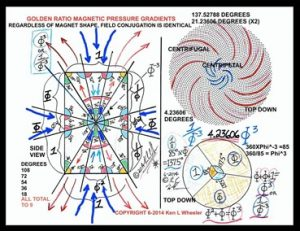As for the 54th Triplet (נית), its value is 460 as in (10 x 46), the value of the word cubit amah (אמה) and its ordinal value is also 46. While this makes its complete value (460 + 46) = 506, like the 32nd Triplet (ושר) and like the value of the key first row (אבגיתץ) of the 42-Letter Name Matrix, the ordinal value and ordinal position of the Triplet is (46 + 54) = 100, whose name in Hebrew is meah (מאה), the permuted form of cubit amah (אמה), which the Ramchal notes is the height of the Holy Temple, 100 cu.

### The Final Letters

Meanwhile, the 46th Triplet (ערי) has the ordinal or conceptual value of 46. Its standard value is 280 as in the 5 final letters (ךםןףץ) in the Alef-bet. While the ordinal value or conceptual value of those 5 final letters (ךםןףץ) is 53, that of the original form of those 5 letters (כמנפצ) is 73, aligning them with the value of the highest accessible dimension, Chochma (חכמה), and the 73rd Triangular Number that represents the Torah’s first verse of 28 letters. The rest of the letters have a total ordinal value of (25373) = 180.

The complete value of the 5 final letters (ךםןףץ) with the kolel is thus (280 + 73 + 5) = 358, Moshiach.

Taking this one step deeper, we see that the collective value of the 5 final letters (ךםןףץ) and their original form 5 letters (כמנפצ) is (3500 + 280) = 3780, as in ““His Name is One” echad ushmo echad (אחד־ושמו־אחד)” and their ratio to the full 27 letters is 3780/4995 = 756.756756…, as in the base of the Great Pyramid.

This also means that the other 17 letters equal 1215, as in the sum of all the elements of Sukkot: The Sukkah (91), the Sach (100), and the 4 Species (1024), and as Rav Abulafia points out, the value of the Hebrew phrase “70 Languages.” And while it is also the value of the first 3 Triplets of the Torah that begin that first verse and which equal 1215, the ratio of the 10 final letters in both their forms to the 27 is 10/27 = .3703703…, which is (.3701 + .0002701) or the values of the 42-Letter Name (3701) and the Torah’s first verse (2701) merged.

The complete value of these 17 (אבגדהוזחטילסעקרשת) standard letters (1215 + 180) = 1395, which equals the gematria value of the 6 Matriarchs—Sarah, Leah, Rivka, Eve, Miriam, and Rachel—whose names’ values are embedded in the 14 Triplets of the 42-Letter Name Matrix, (505, 36, 307, 19, 290, and 238) = 1395. It is also the value of the West Face of the Essential Cube of Creation.

### Lofty Alignment

Another amazing alignment of the 72 Triplet Matrix is with the 10 Commandments and the Spherical Time radius, or Event Horizon. The total value for the 72 Triplets is 9143 and when we square those digits, we get (92 + 12 + 42+ 32) = (81 + 26) = 107, exactly as in Anochi YHVH (אנכי־יהוה) “I am G-d” that begins the 10 Commandments at Chapter 20 and at the 107,007 letter in the Torah. This is also the case for the 67th Triplet (איע) of the 72, which has a complete value is (81 + 26) = 107.

As we said earlier, the third set of 10 integers from 2332 total 275, like the 39th Triplet (רהע) of the 72 Triplets that has a value of 275, as in the cubit and the Primal Frequency (27.5 Hz), with 39 being the value of “G-d is One (יהוה־אחד)” from the Shema and thus from the Torah.  Another aspect to the Matrix of 72 is its coordinate positions, and we find that the 39th Triplet (רהע) has the coordinates row 5, col 7 and when combined with the Triplet (ננא) at row 7 col 5 to form the 5775” height of the Pyramid they have a combined value of (275 + 101) = 376, as in the value of Shalom (שלום), peace, and as in the Spiritual Time radius and pivotal year 3760 HC. Moreover, their combined ordinal values equal 70, which relates to the dating of the Holy Temples that pivot off the date 3760 HC (0 CE).

As for the 55’ entrance height, the sum of those 14 Triplets from Pi (π) found at the 42nd Prime location plus the 14 Triplets of the 42-Letter Name is (11424 + 3701) = 15125 or 5 x 552, with 552 being the measure of the perimeter (3025’) of the Sun and Great Pyramid. The Great Pyramid was designed so that the square root of its perimeter (3025’) dictated its Entrance height (55’ up). It was not chosen just to make entering inconvenient. One would practically have to levitate to reach the one block wide entrance 5.5 stories off the ground, and to do that one would have to understand the elevated use of frequencies.

As explained earlier, the Entrance height was also chosen to align with the two Heis (הה) of Binah and Machut in the Name YHVH (יהוה) and in the Name Ehyeh (אהיה) and in the word for Love, Ahava (אהבה), and for flame (להבה) that have a numerical value of (50 + 5) = 55, the cosmic entrance portal.

While the perimeter of the Great Pyramid is 3025’ or 552,  each of the 4 sides is 756.25’ long or 27.52 ft, and while this is analogous to (42 x 72) = 3024, the value 3025 is equal to the top 10 stacked cubes of the Tower of Truth, the top half, (13 + 23 + 33103) = 3025 cu3, and 55 equals the height of those 10 cubes (1 + 2 + 310) = 55 cu, making the Entrance height of the Great Pyramid quite logical and dynamic for a Pyramid based on and possible encasing the Tower of Truth.

The numerical top half of the Tower represents (55/210) = Phi (φ)2 percent of the Tower, not surprising given that 55 and 21 are the 10th and 8th Fibonacci Numbers, meaning their relationship is necessarily Phi (φ)2. But what is not so obvious and quite ingenious is that the difference between the physical half (50%) of its height and the numerical half of the Tower’s height is (50% – φ2) = (.5055/210) = .238095 = 1/4.2, which brings up the Path of One and the Israelite souls equation 1/(1 + 13 + 91 + 455 + 1820) = 1/(600,000/70/12/3) = 42 = 238.095.

That covers the height and volume of the numeric top half of the Tower of Truth. The area it covers is (12 + 22 +…102) = 385, representing “the Divine Presence of the Creator, the Shechinah (שכינה),” while the cumulative area covered through the top numeric half of that half portion, in order words the top quarter of the Tower, is (12 + 22 +…52) = 55.

The Entrance height to the Pyramid is 55’ high and the gematria of fifty (חמישים) and of five (חמש) together is 756, as in 27.52, the base of the Pyramid around the Tower of Truth, while the 756.25’ when divided by 55 is 756.25/55 = 13.75, which is equal to the circle divided by the Phi(φ)2 angle of the Passage System or 360o/26.18 = 13.75, as in the radiating Phi(φ) angle of rotation (137.5o), but more about that, the wow signal, and more in Part II.